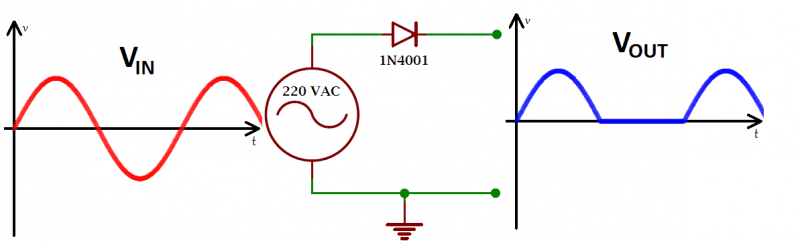# Follow-up question about my understanding on rectification

• nicy12

#### nicy12

I understand the process of rectification but just only in theory. But I still have questions that I guess will be answered if I can experience or has experience about it (but sadly I don't have). Please kindly answer these questions:

1. Does the waveform of Vout can be considered as DC ( having imperfections in it) or it is still an AC?

2. Does the maximum voltage is still 220 VAC in the output waveform or I can apply the theoretical approach wherein there is some drop on LED (about 0.7 V) making the maximum voltage in the output waveform as 219.3 VAC?1. Yes, DC. This is a basic topolgy of a half-wave rectifier
2. Output peak voltage is the same as input peak voltage (220√2 V), but output rms value is smaller.

Q1 - yes you have a DC voltage at the output or ripple voltage. The DC voltage read by a voltmeter is around (√2 * 220V)/Π ≈ 99V
Q2- Without any load Vout = 220V but if you connect a load resistance the output voltage will drop about 0.7 V because the voltage droop across the diode.

Q1 - yes you have a DC voltage at the output or ripple voltage. The DC voltage read by a voltmeter is around (√2 * 220V)/Π ≈ 99V
Q2- Without any load Vout = 220V but if you connect a load resistance the output voltage will drop about 0.7 V because the voltage droop across the diode.

If I add a load of 3k ohms (just for example). Does the output voltage will be sqrt(2)*220/2 - Vdrop of diode - Vdrop of resistor ?

Yes, Vout = √2 * 220V/2 - 0.7V ≈ 154.8V